15.02.04.

PROCEDURE ANALYSIS OF MEASUREMENT OF ELECTRIC POWER CONSUMED BY THE WATER ELECTRIC GENERATOR OF HEAT

Ph.M. Kanarev

E-mail: kanphil@mail.kuban.ru

We have received positive decisions concerning the first applications for issuance of the patents for high efficiency devices generating additional heat energy. Time comes when other investigators join our search. Innovative technology mastering process will move faster if the beginning investigators know the most complicated matters that block commercialization of these devices. That's why we have decided to begin publication of the patented diagrams of the cells of the water electric generator of heat with the description of the most complicated matters that will restrain its commercialization. The measurement procedure of electric power consumed by the water electric generator of heat in household and industry is one of such matters. That's why we analyse this procedure in detail using concrete experimental data.

The measurements have been carried out using a laboratory model of the most effective cell of the water electric generator of heat (Fig. 1). A diagram of this cell will be published when a positive decision or a patent is obtained.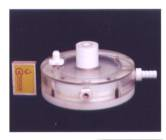Fig. 1. Cell of the water electric generator of heat

A diagram of the experimental assembly is shown in Fig. 2.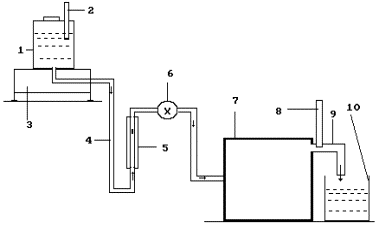Fig. 2. Diagram of the experimental assembly: 1 – a tank for the solution; 2 – thermometer; 3 – electronic scales; 4 – solution feed duct; 5 – rotameter; 6 – solution feed regulator; 7 – cell of the water electric generator of heat; 8 – thermometer; 9 – heated solution drain; 10 – intake tank

Voltage and current at the input of the cell of the water electric generator of heat were measured with the help of two sets of the devices: a voltmeter of 0.2 accuracy class (GOST 8711-78), an ammeter of 0.2 accuracy class (GOST 8711-60) and ACK-20222 electronic oscillograph.

In order to reduce heat losses that were not taken into consideration in the experiment, a solution temperature difference before its heating in the cell and after heating was maintained as not very high: Dt=21°C.

The results of the experiment are given in Table 1.

Table 1

 Indices 1 2 3 Average 1 - mass of the solution, which has passed through the generator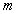,  kg. 0.6 0.624 0.6 0.608 2 - temperature of solution at the input of the generator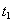, degrees 21 21 21 21.0 3 - temperature of the solution at the output of the generator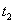, degrees 41 41 41 41.0 4 - temperature difference of the solution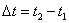, degrees 20 20 20 20.0 5 - durability of the experiment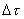, s 300 300 300 300.0 6 - reading of voltmeter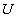, V 5 5 5 5.0 6’ - readings of oscillograph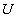’, V 4.8 4.8 4.8 4.8 7 - reading of ammeter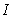, A 0.6 0.6 0.6 0.60 7’ - readings of oscillograph’, A 0.6 0.6 0.6 0.60 8 - electric power consumption according to the readings of voltmeter and ammeter,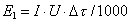, kJ 0.9 0.9 0.9 0.90 9 – heated solution energy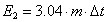, kJ 36.48 37.94 36.48 36.97 10 - COP of the generator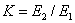40.53 42.15 40.53 41.07

As energy efficiency of the cell proved to be significant, we decided to duplicate the readings of the voltmeter and the ammeter using the oscillograph readings. When we obtained the oscillograms, we applied to a specialist in measuring systems and devices. He was so kind to agree to help us. He processed the oscillograms and sent a method of processing and its results. He advised in his letter that we determined electric power at the cell input correctly. In token of respect we included him as a co-author in the first article where we published the method suggested by him and the results of its application.

As a speciality of measuring systems and devices is rarely met, and its master is a person with a long length of service (he is a pensioner), we have treated him with great respect. All our further activities were based on his authority. The oscillograms of voltage (Fig. 3) and current (Fig. 4) as well as the method of their processing are given below.

Pulse scale is 10. Average voltage amplitude (Fig. 3): Uаср = (29+31+8+33)x10/4 = 252.5 V.

Current magnitude was determined as voltage drop on the resistor with resistance of 0.1 Ohm. Taking this into account, average current amplitude is (Fig. 4):

Iаср = (1.7+0.8+1.7+2.1) x 0.2x10/(4x0.1) = 31.5 А.

Pulse repetition period  Т = 7.4 ms.

Pulse duration tp = 0.28 ms.

Pulse frequency  f = 1000/7,4 = 135.14 Hz.

Relative pulse duration S = 7.4/0.28 = 26.43.

Pulse ratio Z = 0.5/26.43 = 0.019.

Average value of pulse voltage U = 0.019 x 252.5 = 4.80 V.

Average value of current in pulses Iср = 0.019 x 31.5 = 0,60 A.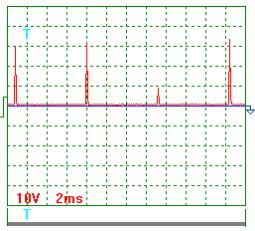Fig. 3. Tension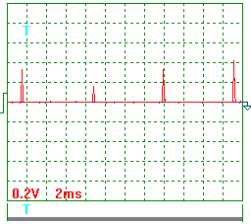Fig. 4. Current

It is clear from these data that the difference between the readings of the voltmeter, the ammeter (Table 1) and the oscillograph do not exceed 5%. It is a reason why we consider the results being obtained as the correct ones.

But later on the author the technique being suggested to us advised that he has doubts concerning correctness of the determination method of energy consumed by the cell of the water electric generator of heat.

As I am a supervisor of studies, I myself have had to carry out a detailed analysis of the measurement procedure of power consumed by the cell of the water electric generator of heat. The results of this analysis are given below.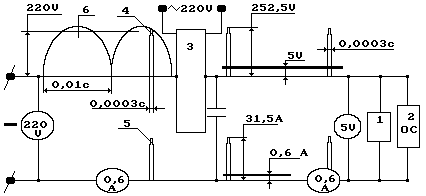Fig. 5. Structural diagram of measurement of electric values of the water electric generator of heat: 1 –cell of the water electric generator of heat; 2 – electric oscillograph; 3 – pulse generator; 4 – voltage pulse; 5 – current pulse; the rest units of the diagram correspond to conventional designations.

In order to facilitate the process of analysis, we had to elaborate a diagram that we called a structural one conventionally (Fig. 5). During the experiment in addition to the data given in Table 1, voltage and current were registered before the pulse generator (Fig. 5, pos. 3). Voltage before the pulse generator was equal to supply-line voltage, i.e. 220 V. Current magnitude remained the same and was equal to 0.60 A.

Average value of voltage of 5.0 V and average value of current of 0.60 A (Fig. 5) at the input of the cell (1) of the water electric generator of heat are written in the diagrammatic presentation of the voltmeter and the ammeter.

As shown in Fig. 5, average amplitude of voltage pulses was 252.5 V with average value of voltage of 5.0 V; average amplitude of current pulses was 31.5 A with average value of current of 0.60 A. Pulse duration was 0.00028 s with relative pulse duration of  26.43 and pulse ratio of 0.019.

In accordance with the readings of the voltmeter, the ammeter and the oscillograph, power at the input of the cell of the water electric generator of heat is P0 = 5 x 0.6 = 3.0 W on the average. Taking this into account, energy efficiency is  41.07 x 100 = 4107% (Table 1). The same result is obtained while processing of the oscillograms.

It is known that a determination of the values of voltage and current in electric circuits with complicated electric pulses (Figs 3 and 4) with the help of the oscillograms is considered to be the most reliable though not the most accurate one. That’s why the oscillograph readings, which differ from the readings of the voltmeter and the ammeter duplicating it by 5%, are considered to be unquestionable. On this basis, a conclusion is drawn that an index of power efficiency of the processes, which take place in the cell of the water electric generator of heat, is nearly 4000%.

In order to be sure that the result being obtained are reliable, it is desirable to duplicate the reading of the voltmeter, the ammeter and the oscillograph with the help of one more, nicer device. An electronic energy meter is the device, but we have no such meter. But we know a principle of its operation, and we can calculate a theoretical result that it is sure to display.

First of all, the electronic meter will register energy of each pulse. As energy is proportional of  pulse area, the electron energy meter will determine area of each pulse of voltage and each pulse of current separately (Figs 3 and 4). Let us assume that it will multiply the obtained areas of pulses of voltage and current by quantity of pulses during one experiment (300/0.0074) = 40540.54. Later on, multiplication of total area of voltage pulses by total area of current pulses should give a value of energy consumed by the cell (1). Taking into consideration a triangular form of the pulses, we’ll have:

voltage pulse area 252.5x0.5x0.00028x40540.54=1418.92;

current pulse area 31.5x0.5x0.00028x40540.54=178.38.

If energy consumed by the generator of heat is registered in such a way, its value will be E = 1418.92 x 178.38 = 253.1 kJ. It is clear that it is an erroneous result, because it is greater than the readings of the devices installed before the pulse generator 220 x 0.6 x 300 = 39.6 kJ. What is the core of the introduced error? In order to find it, let us determine this energy using the pulses of voltage and current for the determination of power being implemented by the cell. For this purpose, it is necessary to find the total area of the pulses of voltage and current per second and to multiply it.  Quantity of pulses per second is equal to pulse frequency of 135.14 Hz. The total area of voltage pulses per second will be 252.5x0.5x0.00028x135.14=4.78 V. The total area of current pulses will be 31.5x0.5x0.00028x135.14= 0.60 A. It is clear that these values coincide with those that have been obtained when processing the oscillograms. Power implemented by the cell will be equal to 4.78x0.60=2.87 W; energy consumed by it from the supply line will be 2.87x300=0.86 kJ. Energy efficiency of the process will be 36.97/0.86=42.50. The voltmeter and the ammeter, which are placed before the cell, confirm this result. In Table 1, this value is equal to 41.07.

Now it is easier for us to find an answer to the question: why do the instruments arranged before the pulse generator (3) indicate that the pulse generator and the cell consume 39.6 kJ in total during the experiment, and the consideration of all pulses of voltage and current gives the value of 253.1 kJ?

In order to give a reply to this question, let us pay attention to the fact that (Fig. 5) current value is the same for the whole circuit, and voltage values are different. Before the pulse generator (3), voltage value is 220 V; after it, before the cell, it is 5.0 V. It appear from this that while calculating energy consumed by the cell by means of area count of pulses of voltage and current we should take overall area of voltage pulses only and multiply this are by current value being the same for the whole circuit, i.e. by 0.6 A. We’ll have 252.5 x 0.5 x 0.00028 x 0.6 x41095.98 = 0.87 kJ. Energy efficiency of the process will be 36.97/0.87=42.50.

Thus, if we record the energy consumed by the cell with the help the electronic energy meter arranged before the cell, we’ll get the same result as the one being obtained due to the readings of the voltmeter, the ammeter and the oscillograph.

Other confirmations that the energy efficiency index of the cell of the water electric generator of heat exceeds 4000% are unnecessary. Under laboratory conditions, this index registration is stable and safe.

A question emerges: can the existing electrical-type instruments arranged before the pulse generator prove this efficiency (Fig. 5, pos. 3)?

It can be early seen (Fig. 5) that with coefficient of performance of the pulse generator being equal or near a unit the ammeter arranged before the pulse generator (3) will indicate 0.60 A and the voltmeter will indicate 220 V. As a result, power implemented by the pulse generator (3) and the cell (1) will be P1 = 220 x 0.60 = 132 W. In this case, energy efficiency of the cell of the water electric generator of heat will be less than a unit (36970/300=123.23)/132=0.93 taking into consideration energy of the heated solution (Table 1).

Thus, even with coefficient of performance of the pulse generator (3) being equal to a unit the readings of the instruments arranged before the pulse generator and after it demonstrate approximately 40fold difference. A question emerges: what instruments give a result corresponding to actual consumption of energy consumed by the cell of the water electric generator of heat? The instruments arranged before the pulse generator (3) or the instruments arranged after it? What is the essence of physics in a difference of these readings?

In order to give an answer to this question, let us consider physical processes that take place while measurement of voltage and current before the pulse generator (3).

The readings of the voltmeter arranged before the pulse generator (3) are greater than the readings of the oscillograph and the voltmeter arranged before the cell (1). It takes place due to the fact that voltage before the pulse generator is always equal to supply line voltage of 220 V.

Let us pay attention to the fact that one pulse (6) of rectified current with duration of 0.01 s is shown in Fig. 5. In the same Fig., a voltage pulse (4) with duration of 0.0003 s is shown. The pulse generator separates it from the whole pulse with duration of 0.01 s and sends it to the cell. Strictly speaking, the remaining area under the curve of a rectified pulse with voltage of 220 V should be reduced by a value corresponding to the area of the pulse that has gone to the cell (1), and it should correspond to voltage 220-5=215 V. But this difference is compensated at once by supply line potential, and this area of pulse with duration of 0.01 s remains corresponding to voltage of 220 V.

Thus, any measuring instruments arranged before the pulse generator (3) will register 40fold greater power than the instruments arranged before the cell (1). Now we know why. Because the instruments arranged before the pulse generator (3) refer current of 0.60 A not to the value of voltage pulse (duration of 0.0003 s), which has gone to the cell of the water electric generator of heat, but to the whole voltage (6) pulse (220 V) with duration of 0.01 s.

Now we can start finding the reply to another question: can the obtained efficiency be implemented under the industrial and household conditions in order to save energy?

As the electric network is a closed loop system, current of 0.6 A will run in this system (with voltage of 220 V) via all electric power meters to a turbine of the power station generator. As a result, all meters will register power implemented by the pulse generator (3) and the cell (1) together, i.e. 132 W. These are the features of electric circuits. We'll get no energy saving.

There are two contradictory results: incontestable energy efficiency of the cell of the water electric generator of heat that is equal to almost 4000% and incapability of the electrical network to accept this efficiency in order to save energy. The main reason of this contradiction is in the fact that the cell consumes energy in the form of short-duration pulses of voltage and current, and there are no such pulses in the electrical network.

What if we forego from the pulse generator and work out an electric power generator that will produce energy in the form of such pulses (Figs 3 and 4), which are consumed by the cell? Such generator should have no electric coupling with the whole electrical network. In this case, power of 3.0 W implemented by the cell will be on the shaft of such generator. If we make this shaft as a common one with the shaft of the electric motor energizing the generator, the common shaft of the electric motor and the generator will give 3.0W to the cell. Power taken by the electric motor from the network will be approximately the same (taking into account the losses).

Conclusion

The voltmeter, the ammeter and the oscillograph arranged before the cell of the water electric generator of heat determine energy, which is consumed by them, in a correct way.

Energy efficiency of the cell of the water electric generator of heat amounting to 4000% is registered persistently and safely under the laboratory conditions.

High energy efficiency of the water electric generator of heat can be implemented in modern electrical networks via an individual generator producing electric energy in the form of pulses of voltage and current consumed by the water electric generator of heat.

References

1. Kanarev Ph.M. The Foundations of Physchemistry of Micro World. The Third Edition.  2003. (In Russian). http://Kanarev.innoplaza.net

2. Kanarev Ph.M. The Foundations of Physchemistry of Micro World. The Fourth Edition. 2004 (in English). http://Kanarev.innoplaza.net

3. Ph.M. Kanarev. Prediction of Evolution of Fundamental Physical Investigation. 2004. 160 pages. (In Russian). http://Kanarev.innoplaza.net  Book 2.

Webmaster:

Suggested reading for experimenters wanting to repeat the ‘Kanarev Effect’:
Part 4 (2.3 Mb) of "The Foundation of Physchemistry of Micro World", the Fourth edition.

Procedure Analysis of Measurement of Electric Power
Consumed by the Water Electric Generator of Heat
by Prof. Kanarev:  http://Kanarev.analysis.innoplaza.net

<< Kanarev´s Page